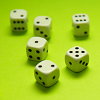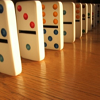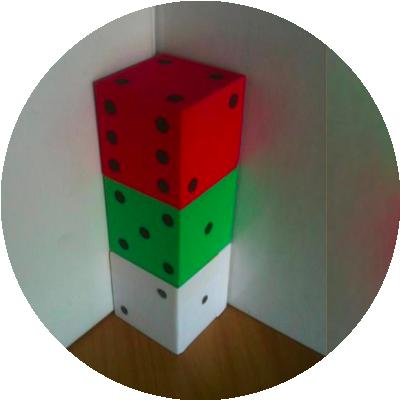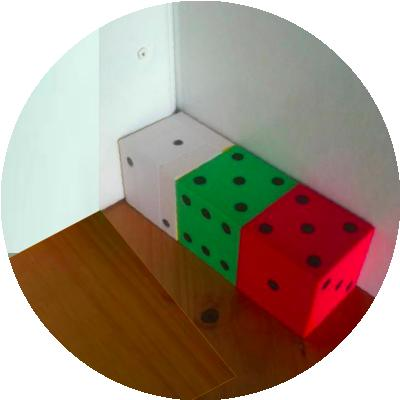#### You may also like### Consecutive Numbers

An investigation involving adding and subtracting sets of consecutive numbers. Lots to find out, lots to explore.### Roll These Dice

Roll two red dice and a green dice. Add the two numbers on the red dice and take away the number on the green. What are all the different possible answers?### Domino Square

Use the 'double-3 down' dominoes to make a square so that each side has eight dots.

# Dice in a Corner

## Dice in a CornerThere are three dice sitting in the corner with the simple rule that where two dice meet there must be the same numbers facing each other.
So, in the first picture above there are $3$'s at the bottom of the red dice and on the top of the middle green and there are $4$'s on the bottom of the green dice and the top of the white dice. The numbers on the seven faces that can be seen are then added and make $21$.

In the second picture above there are $4$'s at the left of the red dice and on the right of the green dice and there are $3$'s on the left of the green dice and the right of the white dice. The numbers on the seven faces that can be seen are then added and make $23$.

#### Your challenge is to arrange dice (using at least $2$ and up to as many as you like) in a line from the corner, so as the faces you can see add up to $18$ (instead of the $21$ and $23$ above), in as many ways as possible.

Each line of dice must be along or up a wall (or two walls).  A line going up is counted the same as a line going along. Remember the dice must touch face to face and have the same numbers touching. The lines of dice must be of a single thickness, so this one below is not allowed;### Why do this problem?

This activity engages the pupils in both a spatial and numerical context and gives them the freedom to choose how they go about it, for example visualising in their head, using dice  and/or making use of a spreadsheet. They can learn a lot from adopting one method and then realising that an alternative method would be better.  This particular activity also lends itself to team work.

### Possible approach

It would be advisable to use a corner with large dice to set up the challenge.  (The corner in the pictures was made from three pieces of card.)  It is useful to have three different colours for the dice so that it is easier for the pupils to say which dice they are talking about.

Demonstrate the rule of "like faces facing", and then move the dice around a bit so that the rule is broken and ask if it's ok. Put the dice in the arrangement in the pictures and clarify how the total is calculated.

Before setting the children off on the challenge itself, you could ask them to find the smallest and largest possible totals for a stack of three dice so that they become immersed in the context.

### Key questions

Open questions such as: